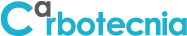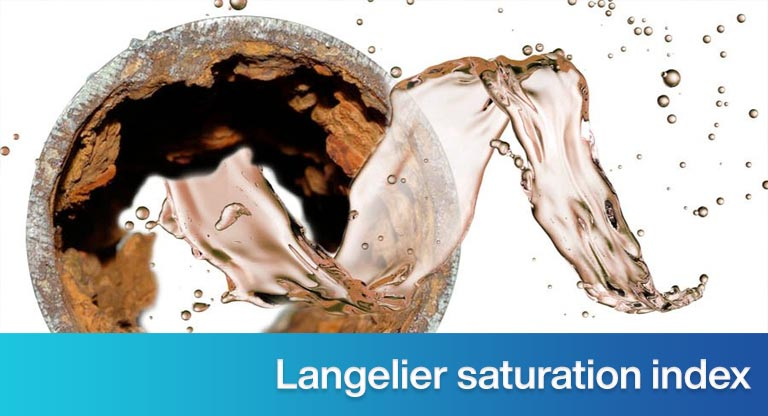search
0Cerrar ventana

# The fouling, corrosive or balanced tendency of a water, and the Langelier saturation index

##Índice del contenido

## Langelier Saturation Index.

To avoid problems in its handling and treatment, the ideal is that a water is balanced.

A corrosive water affects the pipes and iron equipment.

Encrusting water, precipitates on most surfaces. Some, such as polyethylene and polypropylene, suffer less fouling, because the salts that precipitate do not anchor in them efficiently.

If a system does not have iron parts, the corrosivity of the water is no longer a problem. However, if a water is encrusting, it is a problem in the whole system. If we treat fouling water through typical purification processes, it affects everyone. It affects filters (granular media, cartridges, discs, bags or others), although to a lesser degree. Encrusting water:

• It precipitates on the walls of the activated carbon, and this stops performing its adsorbent or oxidant elimination function.
• It precipitates on the walls of the resins and they stop exchanging ions.
• They precipitate in the quartz tube of the UV lamps and they stop disinfecting.
• They precipitate on the reverse osmosis membranes and plug them.

The tendency of a water to be encrusting, corrosive or balanced, depends on three parameters:

• pH
• Total alkalinity
• Hardness

The lower the value of any of the three above, the less encrusting (or more corrosive) water becomes.

Total dissolved solids do not influence the fouling or corrosive tendency of the water, but they do potentiate said tendency, because they increase the conductivity of the water. Another parameter that potentiates the tendency of water is its temperature: the higher the temperature, the tendency increases, because the viscosity decreases and the mobility of the ions (with their respective electrons) increases.

From the above, it is possible to decrease the fouling tendency of a water:

• Lowering the pH
• Decreasing total alkalinity
• Decreasing the total hardness

There is no exact way to calculate the fouling or corrosive tendency of a water, but there are correlations that allow estimating this tendency.

The pH is lowered, adding a protonic acid.

The alkalinity is lowered (a) by adding an acid; or (b) exchanging it for another anion (using ion exchange resins).

The hardness is lowered, softening the water by means of sodium cycle ion exchange resins.

If adding an acid decreases the fouling tendency of a water, why not just do it like this? There are two reasons:

• Due to the danger of strong acids, which are the cheapest and most effective in reacting with alkalinity.
• Because of how difficult it is to control the amount of acid to add, to reach the desired pH.

It is possible to reduce the corrosive tendency of a water:

• Increasing the pH
• Increasing total alkalinity
• Increasing the total hardness

The pH is increased by adding a hydroxide (such as soda or potash).

Alkalinity is increased by adding to the water a carbonate (such as Calcite, which is calcium carbonate), a bicarbonate or a hydroxide (such as Corosex, which is magnesium oxide, which when in contact with water hydrolyzes and dissolves as ion Mg+2 and OH ion–).

The hardness is increased, adding it to the water (such as through Calcite or Corosex).

Langelier saturation index

There are various indices with which the corrosive or encrusting tendency of a water is estimated: Langelier, Ryznar, Larson and Duswell, Riddick, Mc Cauley, Caldwell-Lawrence …

Each one better predicts the behavior of water under certain conditions.

The Langelier index, or Langelier saturation index (ISL) is the most used. For a particular water, the ISL is calculated:

ISL = pH – pHs

Where pH is the value of said water and pHs It is the pH value at which it would be at saturation.

A Langelier saturation index with a value of zero corresponds to balanced water. A positive value corresponds to encrusting water and a negative value corresponds to corrosive water. The further away the value is from zero, the greater the trend.

It is little more than impossible to have a water with a Langelier Index of zero. Water can be considered balanced when its value is between -0.3 and +0.3.

 Langelier saturation index value Water trend +0.3 to +2.0 High fouling. 0.0 to +0.3 Light scale with corrosion. 0.0 Balanced. Light corrosion can occur. 0.0 to -0.3 Light corrosion. Scale does not form. -0.3 to -2.0 High corrosion.

PHs it depends on the total alkalinity, total hardness, total dissolved solids and the temperature of the water in question. It is calculated through graphs, tables or correlations published by various authors. Some websites have calculators available to do this.

Corrosion Doctors publishes the following correlation:

pHs = 9.3 + A + B – C – D

In which:

A = (log SDT – 1) / 10

B = 34.55 – (13.12 log T)

C = log DC – 0.4

D = log AT

In the above expressions,

TDS = concentration of total dissolved solids, in mg / L

T = temperature, in ° K

DC = calcium hardness, in mg CaCO3/ L

AT = total alkalinity, in mg CaCO3/ L

Example:

What is the value of the Langelier saturation index of a water with the following parameters: pH = 8.8, SDT = 320 mg / L, DT = DC = 150 mg CaCO3/ L (all total hardness is calcium), AT = 34 mg CaCO3/ L and T = 25 ° C? How corrosive or encrusting can you expect this water to be?

Calculation of the Langelier saturation index:

T = 25 ° C = 298 ° K

A = (log SDT – 1) / 10 = [log (320) – 1] / 10 = 0.15

B = 34.55 – (13.12 log T) = 34.55 – (13.12 log 298) = 34.55 – 32.46 = 2.09

C = Log DC – 0.4 = Log (150) – 0.4 = 1.78

D = Log AT = Log (34) = 1.53

pHs = 9.3 + A + B – C – D

pHs = 9.3 + 0.15 + 2.09 – 1.78 – 1.53

pHs =  8.2

ISL = pH – pHs  = 8.8 – 8.2 = 0.6

This water can be expected to be very encrusting.

Another example:

If the water in the previous example is softened, what Langelier Index is already softened?

Calculations:

When softening, the hardness is exchanged for sodium.Now you have a water with the following variables:

pH = 8.8.
TDS = 329 mg / L
DT = DC = 0
AT = 34 mg CaCO3/ L
T = 25 ° C

Calculation of the Langelier index:

T = 25 ° C = 298 ° K

A = (log SDT – 1) / 10 = [log (329) – 1] / 10 = 0.15

B = 34.55 – (13.12 log T) = 34.55 – (13.12 log 298) = 34.55 – 32.46 = 2.09

C = Log DC – 0.4 = Log (0) – 0.4

There is no logarithm of zero, but we can assume a low value of DC. Say, 0.01:

C = Log DC – 0.4 = Log (0.01) – 0.4 = -2.4

D = Log AT = Log (34) = 1.53

pHs = 9.3 + A + B – C – D

pHs =  9.3 + 0.15 + 2.09 – (-2.4) – 1.53

pHs =  12.4

ISL = pH – pHs  = 8.8 – 12.4 = -3.6

The water becomes extremely corrosive.

### References.

¿La información fue útil?

Artículo anterior

#### Silver

Artículo siguiente

Llamar